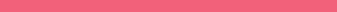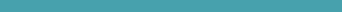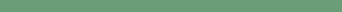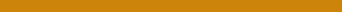Enable javascript in your browser for better experience. Need to know to enable it? Go here.
•Australia
•Brazil
•Canada
•Chile
•China
•Ecuador
•Germany
•India
•Singapore
•Spain
•Thailand
•United Kingdom
•United States
•Worldwide

# Regression

A traditional approach to analyzing data that aims to create models from past behavior that can be used to make future predictions.

A statistical approach to data analytics that attempts to determine the relationship between a set of independent variables.What is it?

A statistical approach to data analysis, where you create models for relationship between certain variables, such that you can make predictions.What’s in it for you?

Regression is a relative inexpensive and understood approach to making future business decisions, based on data.How is it being used?

Many demand forecasting tools use regression analyses. It’s often used in economics and finance.

### What is it?

Regression is a statistical approach to modeling data. There are a number of different types of regression models — linear, polynomial, non-linear etc. — which attempt to define patterns between different variables.

It can be useful for identifying a pattern in the data that allows you to spot trends. For instance, a sales manager trying to predict next month’s figures might use regression analysis to work out, using previous sales data, the likely impact of the summer weather.

### What’s in for you?

Regression analysis is one of many traditional statistical methods, which are well understood and relatively inexpensive, that can be used to make predictions.

### What are the trade offs?

Essentially, regression works by using data to plot a graph and trying to fit a line that describes the relationship between the variables. In many cases, the behavior being modeled can be more complex than your fitted line suggests. So regression may be too simplistic for the behavior you’re trying to predict.

If your model is wrong, you may need a more sophisticated approach. You’ll essentially need to train more complex models.

### How is it being used?

Regression is best suited to relatively simple prediction problems such as demand forecasting, or pricing. If your business can be run from a spreadsheet, regression might well be a good fit for you.